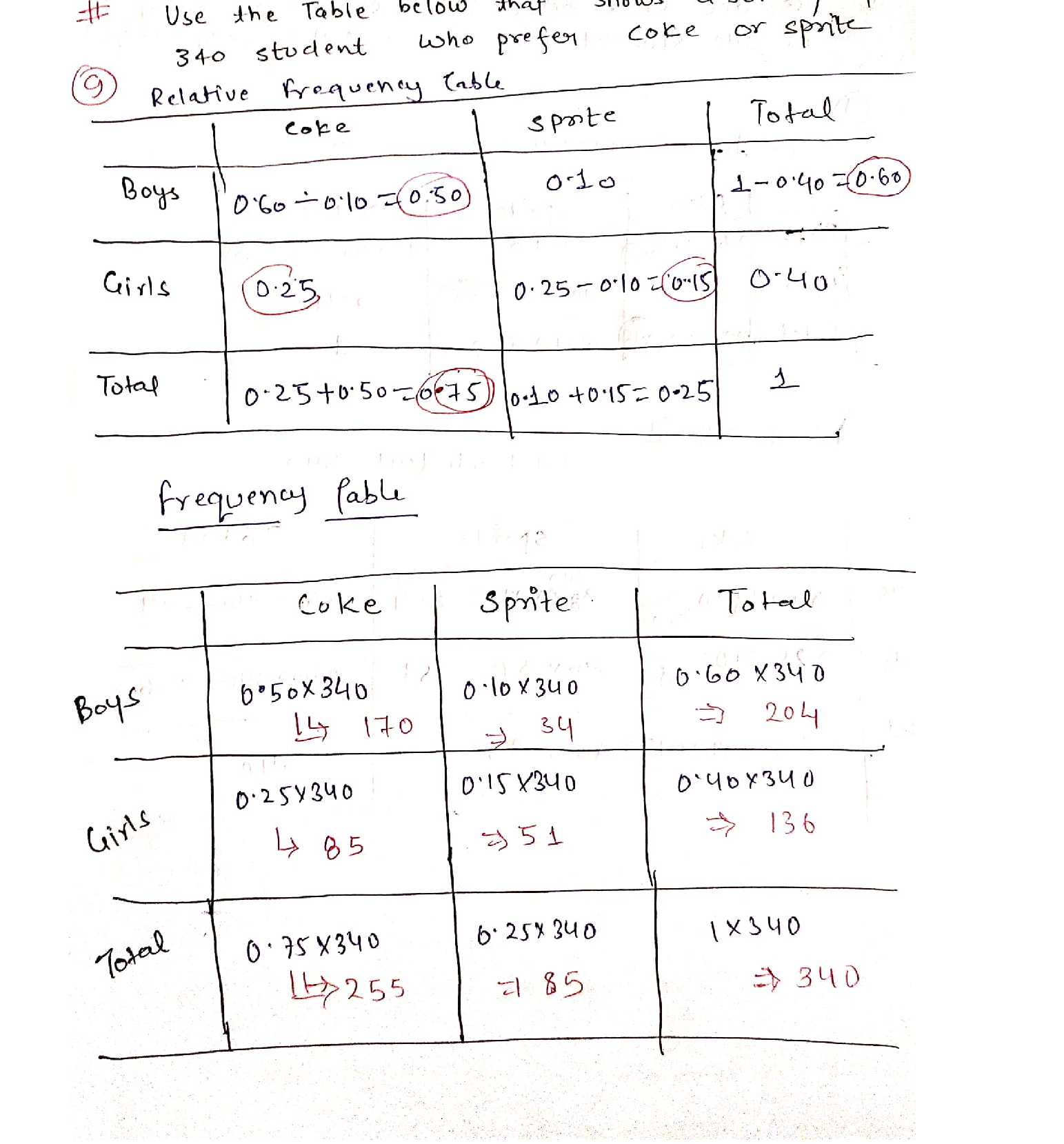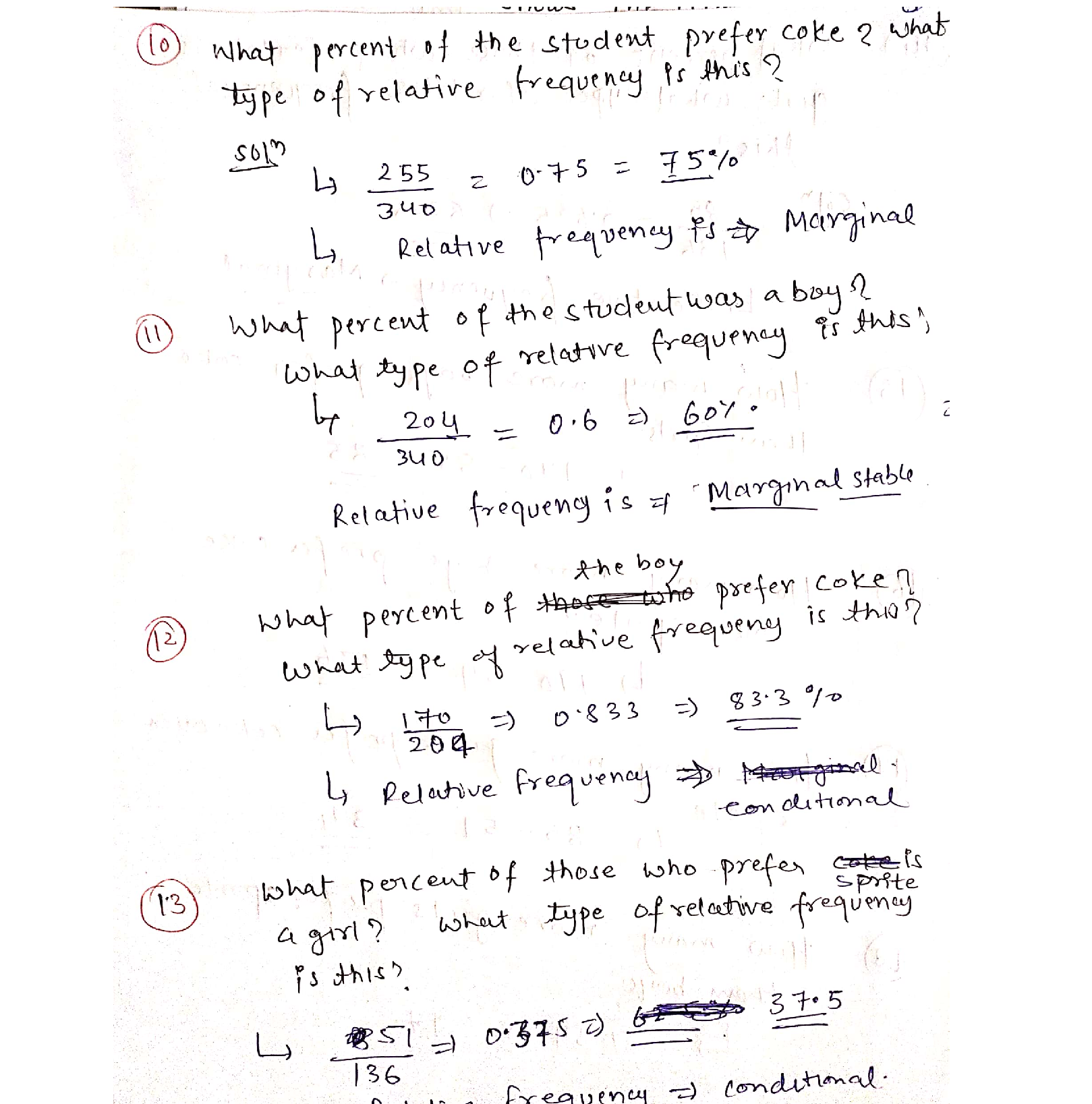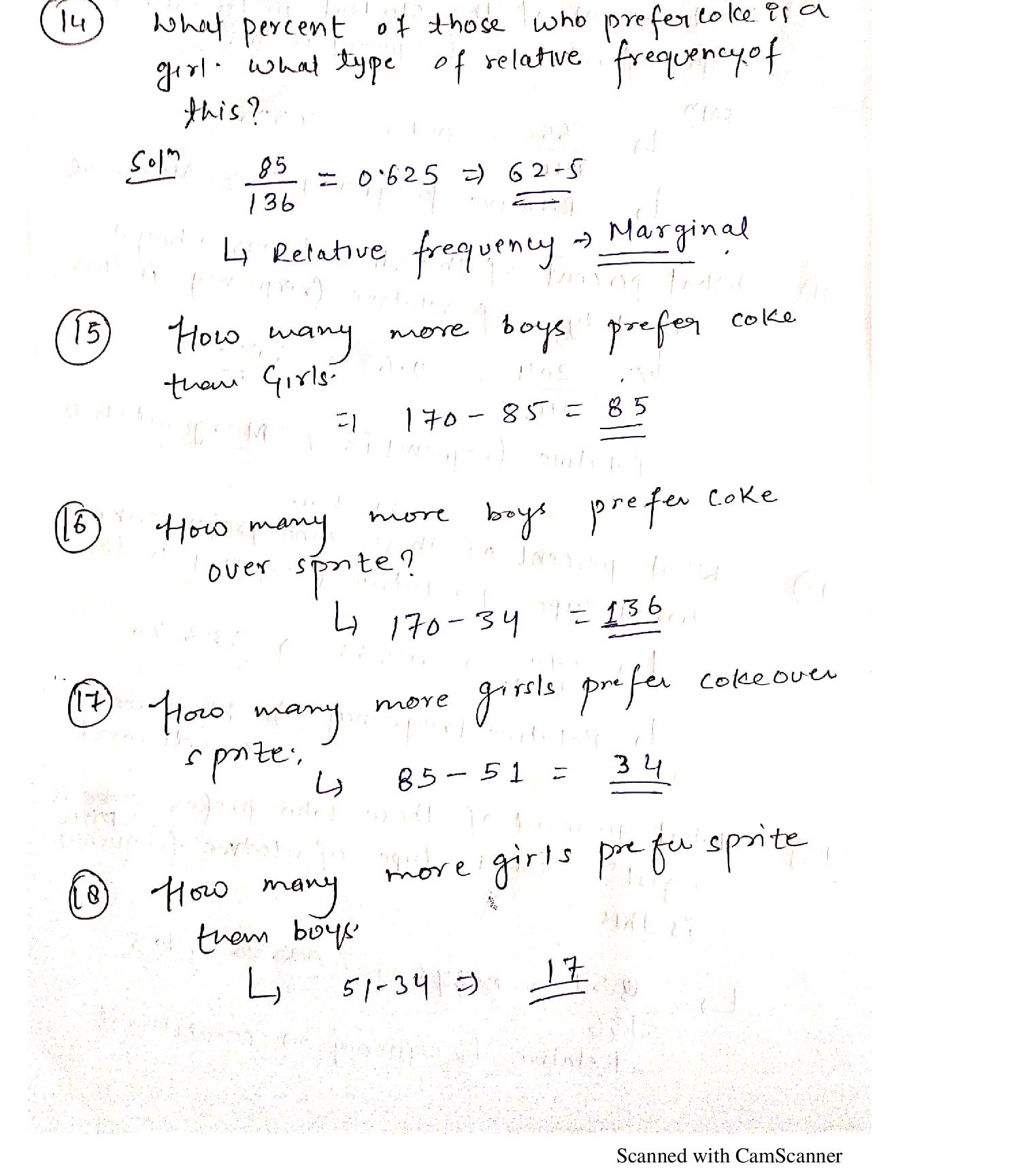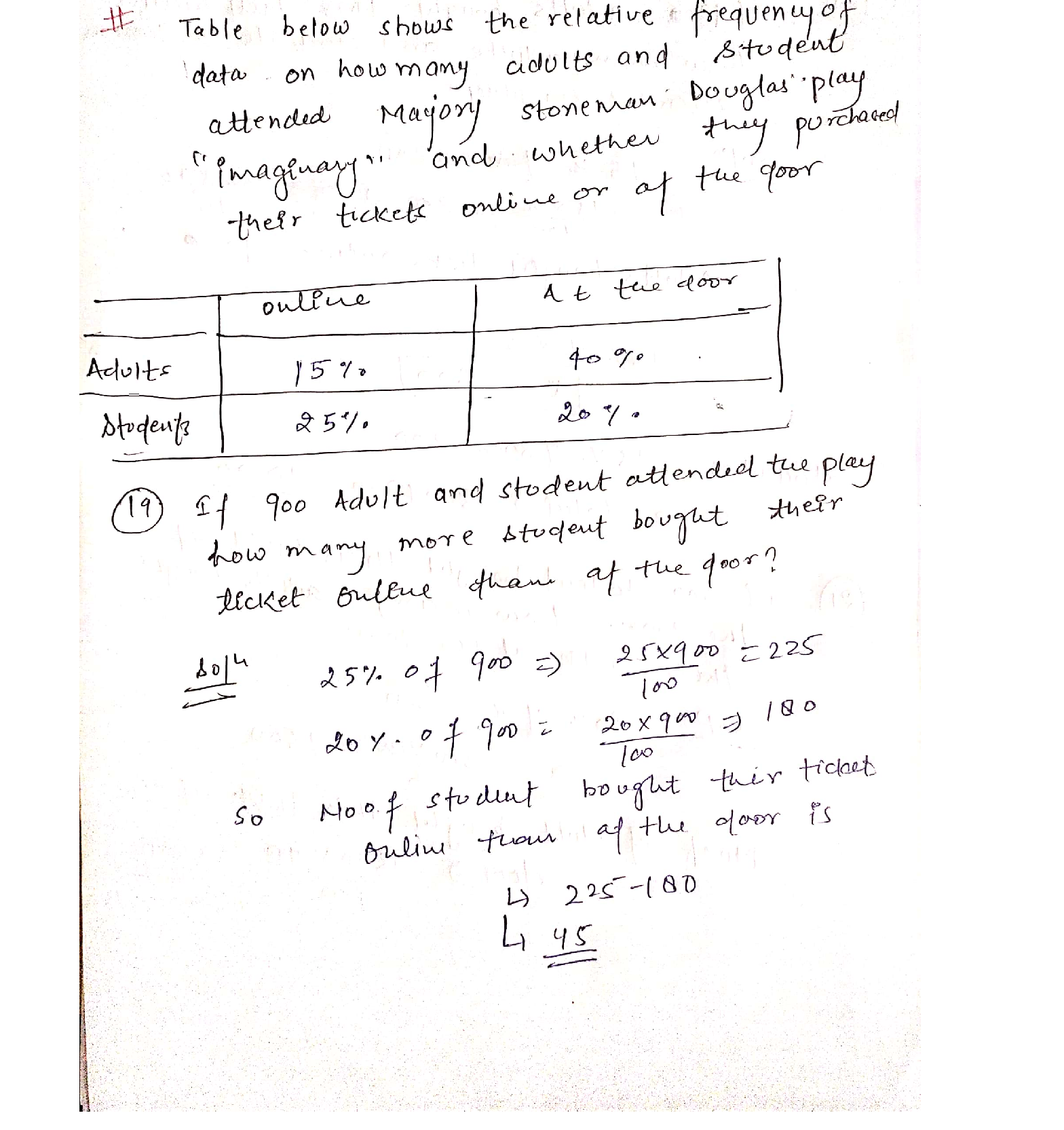Question

Linear Algebra

Use the table below that shows a survey of 340 students who prefer Coke or Sprite.

9.Complete both tables. The top is the relative frequency table, and the bottom is the frequency table.

10. What percent of the students prefers Coke? What type of relative frequency is this?

11. What percent of the students was a boy? What type of relative frequency is this?

12. What percent of the boys prefers Coke? What type of relative frequency is this?

13. What percent of those who prefer Sprite is a girl? What type of relative frequency is this?

14. What percent of those who prefer Coke is a girl? What type of relative frequency is this?

15. How many more boys prefer Coke than girls?

16. How many more boys prefer Coke over Sprite?

17. How many more girls prefer Coke over Sprite?

18. How many more girls prefer Sprite than boys?Verified### Question 52404Linear Algebra

In an election campaign, the popularity, B, as a percent of voters, of the governing Blue party can be modelled by a function of time, t, in days throughout the campaign as B(t) = 40 - 0.5t. The popularity, R(t), of the opposing Red party can be modelled by a composite function of B(t),R(B(t)) = 20 + 0.75[40 – B(t)].
Graph B(t) and describe the trend.

### Question 45665Linear Algebra

\text { 4] Find } g(0)-g(9)+g(2) \text {, if }
g(x)=\left\{\begin{aligned} \frac{x+1}{2}, & \text { if } x \text { is odd } \\ \frac{x}{2}, & \text { if } x \text { is even } \end{aligned}\right.

### Question 45664Linear Algebra

\text { 3] Find } f(4)-f(2)+f(3) \text {, if }
f(x)=\left\{\begin{array}{ll} \frac{x+1}{2}, & \text { if } x \text { is odd } \\ \frac{x}{4}, & \text { if } x \text { is even } \end{array}\right.

### Question 45663Linear Algebra

2] Find the Domains of the following functions:
\text { a) } f(x)=\sqrt{15-5 x}
f(x)=\frac{x^{2}-2 x+1}{x^{2}-4 x-21}
f(x)=\frac{x^{2}-2 x+1}{\sqrt{16-2 x}}

### Question 45662Linear Algebra

a) Give the definition of a rational function. [5 pts]
b) Give an example of a polynomial function of degree 3. [5 pts]
c) Can a constant function be a polynomial and a rational function at the same time? Explain your answer. [5 pts]
d) Give an example of a non-polynomial function and explain why not apolynomial function. [10 pts)

### Question 45333Linear Algebra

5) Ве,
\begin{array}{c} f: \mathbb{R} \rightarrow \mathbb{R} \\ f(x)=\left\{\begin{array}{ll} x^{2}-3 \cos (\pi x) & x<0 \\ x-4 e^{-2 x} & x \geq 0 . \end{array}\right. \end{array}
Calculate
\int_{-1}^{2} f(x) d x
Presenting the result in simplified form

### Question 45332Linear Algebra

4) Be f: R - Ra function differentiable in R such your derivative f', has in maximum a real zero.
Prove that the equation f(x)=0 has in maximum 2 real square

### Question 45331Linear Algebra

3) Take in consideration the following function
f(x)=\left\{\begin{array}{ll} f: \mathbb{R} \rightarrow \mathbb{R} & \\ \frac{x^{2}-4 x+\cos (\sin (x)),}{x^{4}+4 x^{2}+1}, & x \leq 0 \end{array}\right.
a) Show that the function f is continuous in R+ and in R- but discontinuous in the point X
b) Say justifying, if f is differenciable in X=0

### Question 45330Linear Algebra

2) Prove by definition that
\lim _{x \rightarrow 0} x^{2} \cos \left(e^{x}\right)=0

### Question 45329Linear Algebra

1) Calculate the following limit:
\lim _{x \rightarrow+\infty} \frac{x^{2}\left(e^{-3 x}+1\right)+x \cos (5 x)}{x^{2}+7 x+1}

### Submit query